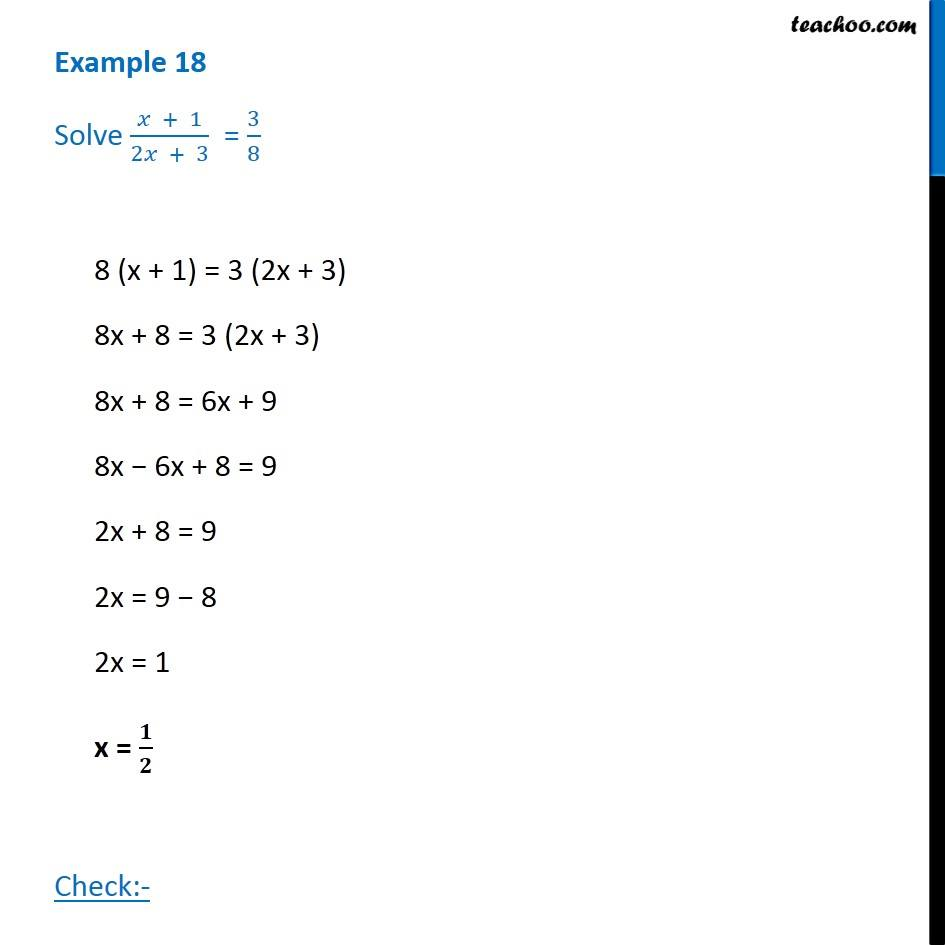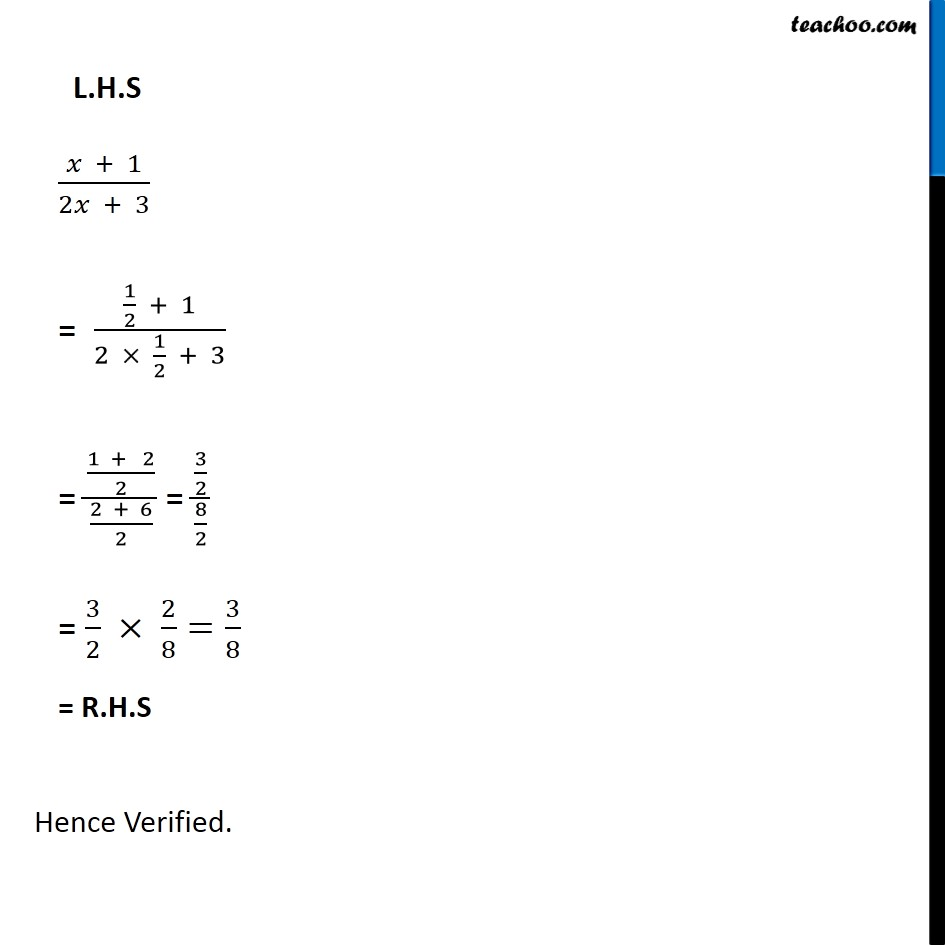Examples

Chapter 2 Class 8 Linear Equations in One Variable
Serial order wiseLearn in your speed, with individual attention - Teachoo Maths 1-on-1 Class

### Transcript

Question 14 Solve (𝑥 + 1)/(2𝑥 + 3) = 3/88 (x + 1) = 3 (2x + 3) 8x + 8 = 3 (2x + 3) 8x + 8 = 6x + 9 8x − 6x + 8 = 9 2x + 8 = 9 2x = 9 − 8 2x = 1 x = 𝟏/𝟐 Check:- L.H.S (𝑥 + 1)/(2𝑥 + 3) = (1/2 + 1)/(2 × 1/2 + 3) = ((1 + 2)/2)/((2 + 6)/2) = (3/2)/(8/2) = 3/2 × 2/8=3/8 = R.H.S